## WE ARE OPEN | SERVICE, TECH SUPPORT, PARTS, FAST LEAD TIMES FROM 6 LOCAL PLANTS

### Rep Our Products

Are you interested in representing CaptiveAire and selling our products?
Please fill out the following form, and we will contact you soon.

0 / 500
0 / 500
0 / 500
0 / 1000

PrintAdobe PDF Version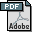Fan Applications & System Guide

Ventilation Calculation Methods

Airflow for general ventilation can be calculated by;
• Area Method
• Air Change Method
• Occupancy Method
Area Method – Derives the ventilation rate from the area of the space (in square feet) to ventilated multiplied by ventilation rate per square foot.
Example: For residential bathrooms up to 100 sq. ft. in area, HVI recommends an exhaust rate of 1 CFM per square foot. A bathroom is 6’ x 10’ or 60 sq. ft.

Multiply 1 cfm x 60 sq. ft. for a flow rate of 60 cfm.
Air Change Method - Derives the ventilation rate from the volume of the space (in cubic feet) to be ventilated multiplied by the number of total air changes in one hour.
Example: For an auditorium the suggested air change rate is 4 to15 air changes per hour. An auditorium is 80’ x 90’ with 20’ ceiling or 144,000 cu. ft. Use 10 air changes per hour.

 CFM = 144,000 cu. ft. x 10 AC p/ hr CFM = 24,000 60
Occupancy Method - Derives the ventilation rate from the number of people that will occupy the space at any given time.
Example: For an office, the recommended ventilation rate is 20 cfm per person. The occupancy of a general office is one person per 80 to 150 sq. ft. An office is 40’ x 60 ‘ or 2,400 sq. ft. Occupancy = 2,400 sq. ft./150 sq, ft p/ person

CFM = 16 people x 20 cfm per person     CFM = 320
Heat Removal Method
When the temperature of a space is higher than the ambient outdoor temperature general ventilation can be used to provide cooling. What is needed to calculate CFM is the amount of heat to be removed in BTU/hr, the desired indoor temperature and design outdoor dry bulb temperature.
Example: 200,000 BTU/hr to be removed, 70 degree desired indoor temperature and 90 outdoor dry bulb temperature.

CFM = 200,000 (BTU/hr) / (1.08 x 90 - 70)     CFM = 9,260
(Note: rule of thumb outdoor / indoor temperature differential is 20 degrees)

Applications

Listed below are ventilation rates for some common applications. Where more than one method is shown use the method that results in the higher airflow rate.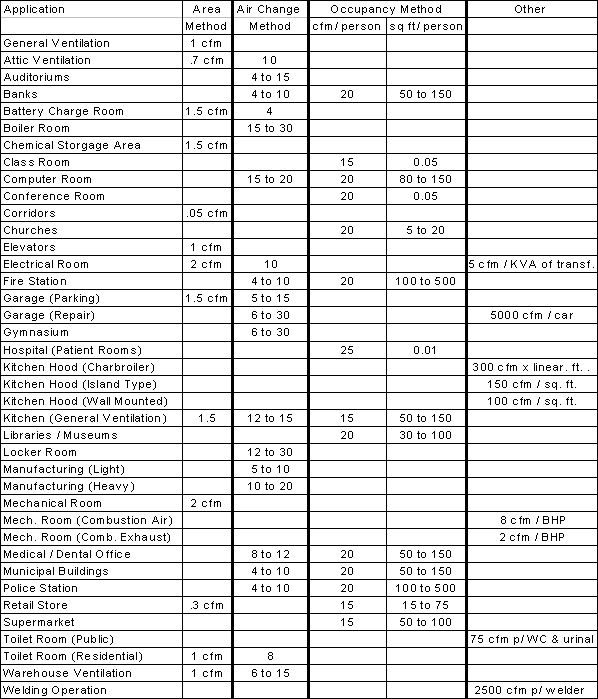Systems
General Ventilation
• Plan and Spec Jobs - required airflow and system static pressure is calculated by the engineer and is listed on the fan schedule.
• Retro Fit and Design Build Jobs - the system static pressure can be calculated using the required airflow, the duct size, duct layout and total duct length and duct friction loss chart (Friction Loss Chart needed).
• When the duct layout is not known the following rules of thumb can be used to estimate system static pressure
1. Non Ducted Applications - .10” to .25”
2. Ducted Applications - .20 to .40 per 100 feet of duct (based on duct velocity of 1000 to 1800 feet per minute). Fittings .08” per fitting (elbows, register, grille, damper)
Example: Retrofit a fan for a mechanical room. Area of room is 20’ x 60’ or 1,200 sq, ft. Fan is ducted to roof with 16” x 16” duct. Total duct run is 25’. There are 2 elbows and a grille in the system.

Required Air Flow - 2,400 cfm. (2 cfm p/ sq. ft. x 1200 sq. ft.)
Duct Velocity – 1,400 fpm (air flow / duct area)
Duct Losses - .08” (.30” / 100 ft of duct x 25’)
Fitting Losss - .24” (.08” x 3 fittings)
Estimated SP. = .08” + .24”, round to nearest 1/8”. Size Fan for 2,400 cfm @ .375” sp

Another rule of thumb that can be used if number of fittings is not known is;
 Estimated SP. = 1.5 x System Length (ft.) x Friction Rate (Inches W.G.)* 100 100
*Friction Loss Chart needed

Kitchen Hoods
• Use .625 to 1.50” sp.
• Minimum duct velocity 1,500 fpm
• Make up air 80% of exhaust air

Sound Level Guidelines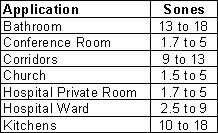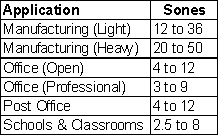Basic Fan Laws
Fan Laws are equations used to predict a fan’s performance at other conditions. Once a system is installed if the CFM, SP and BHP are known (by measurement) the CFM, SP and BHP at every other point along the system curve can be calculated.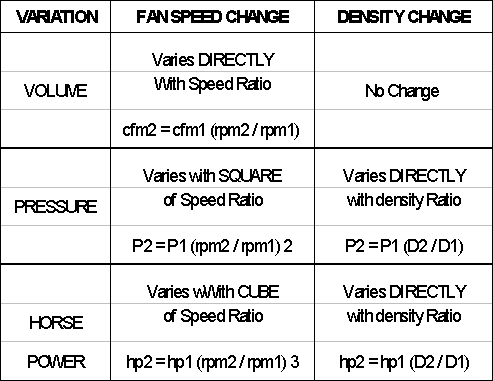Example: Fan is selected at 4,000 CFM @ .50” SP, 825 RPM and .72 BHP. Balancer reports 3,000 CFM @ .375” SP, 825 rpm and motor operating at nameplate full load amps.

Calculate the required RPM using fan laws
CFM2 / CFM1 = RPM2 / RPM1
4,000 / 3,000 = RPM2 / 825, New RPM = 1100
Calculate the new SP using fan laws
SP2 / SP1 = (RPM2 / RPM1)2
SP2 / .375” = (1100 / 825)2 , New SP = .67
Calculate the new BHP using fan laws
BHP2 / BHP1 = (RPM2 / RPM1)3
BHP2 = .75 because fan motor was operating nameplate amps
BHP2 / .75 = (1100 / 825)3 , New BHP = 1.78

The system SP is actually higher than calculated. To meet the operation point at the new SP the fan will need to operate at 1,100 rpm and will require a 2 hp motor.

Air Density Correction
Fan performance tables are based on standard air conditions. An installation’s altitude and the air temperature will affect the air density. Use the Air Density Correction Factors (df) listed below to determine the corrected fan performance. Correction for temperatures between 40 deg. F and 100 deg. F and/or elevations between –1,000 ft. and +1,000 ft. are generally not required.

Air Density Correction Factors (df)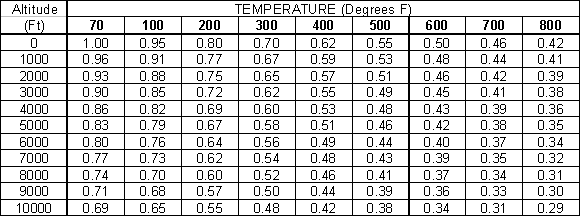Example: A fan is selected to deliver 4,000 CFM @ .25” SP. Fan will be installed 4,000 ft above sea level; air temperature is 70 deg. F. From the Air Density Correction Factor table above the density correction factor (df) is determined to be .86 by using the fans operating altitude.

Divide design SP by the Air Density Correction Factors (df) .25”sp / .86 = .29” sp

It is determined by from the fan performance table that the fan must operate at 774 rpm to develop the design CFM at the corrected SP at 4,000 feet above sea level at the operating temperature of 70 deg. F.

From the fan’s performance table it is determined that at standard conditions .51 BHP is needed meet the design point. This is corrected to conditions at altitude by multiplying the BHP by the Air Density Correction Factor

.51 BHP x .86 = .44 BHP

The final operating conditions at altitude are determined to be 4,000 CFM @ .25” SP, 774 rpm and .44 BHP